May 3, 2019

# One-shot object detection

We know that a convolutional neural network is used to predict what object appears in some image, at the end of the network we have a dense layer with the softmax or sigmoid activation function with n neurons for n classes, besides the object prediction we can also use a neural network to predict the coordinates of this object.

There are multiple models which are good to predict coordinates like SSD, YOLO or SqueezeDet, we call these models

one-shot detectors. Since SSD and YOLO v3 work generally in the same way in this post we will see how a one-shot detector based on these two models works.

## One-shot detector

To build our one-shot detector we can use any architecture like the Mobile net model, this model will extract the features of the image like a normal neural network does, however we will load the model without the last dense layer (or fully connected layer), our one-shot detector will not use dense layers but convolution layers instead.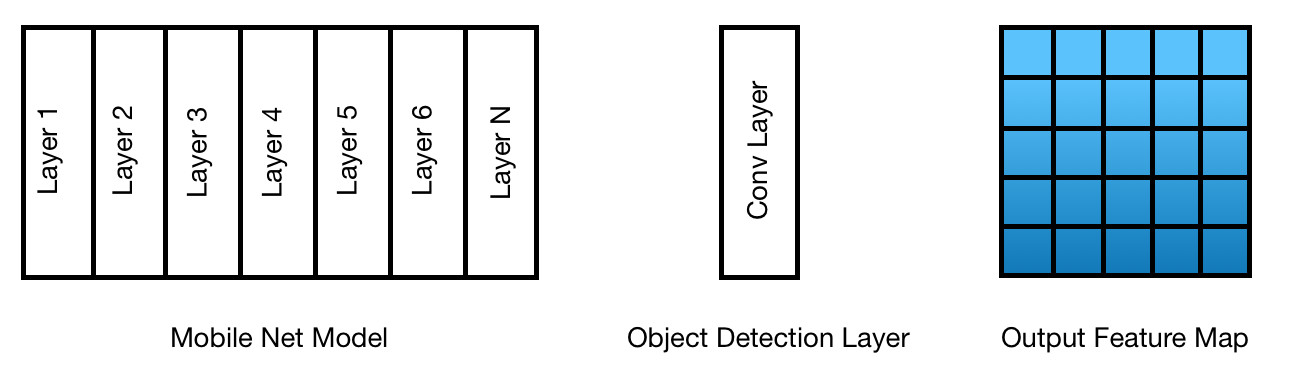Image A

In TensorFlow we can create the model as follows:

``````
def create_model():

## Mobile Net model

mobile_model = MobileNetV2(

input_shape=input_img_size,

include_top=False)

mobile_model_output = mobile_model.output

## Object Detection Layer

feature_map = Conv2D(5, 3, padding='valid', activation='sigmoid')(mobile_model_output)

model = Model(inputs=mobile_model.input, outputs=feature_map)

return model

``````

include_top=False will load the model without the last dense layer. We can also notice that our last layer is a convolutional layer with 5 kernels of size 3x3, with this we will obtain an output feature map of size 5x5x5.

With the simple code above we have our one-shot detector network ready, actually the hard work behind this detectors is building the labels variables (y_train, y_val) as well as the loss function.

In a normal architecture the output of the last dense layer is a probability distribution for each class, if we have 2 classes the output would be an array of 2 values which sum to 1:

``````
predicted_classes = [0.2, 0.8]

``````

And we would also need the labels array for that particular image to compute the loss function:

``````
y_true = [0, 1]

``````

In our one-shot detector network we have a feature map as output, in the image A we have an output feature map of size 5x5x5, since each cell in the feature map has one detector, in total we have 25 detectors and each detector will search for an object in its specific position, in fact this feature map is a 3d tensor, the first 2 dimensions indicates how many cells the feature map has and the last dimension has cs + 4 + n values where n is the number of classes, cs is the confidence score and the other 4 values are the coordinates x_min, y_min, x_max, y_max of the object, the confidence score will tell us how sure the model is that in that detector there is an object, for example if we want to classify [people, cars, bicycles] the array could be:

``````
[0.7, 0.34, 0.25, 0.64, 0.67, 0.24, 0.026, 0.734]

``````

This means that the model is 70% sure that there is an object in this position, the coordinates of this object are x_min=0.34, y_min=0.25, x_max=0.64, y_max=0.67 and finally that the object is a car since [0.24, 0.734, 0.026] the second position of the classes scores has the biggest value, as a result we will have 25 arrays of this type, each one will search for an object in a certain position.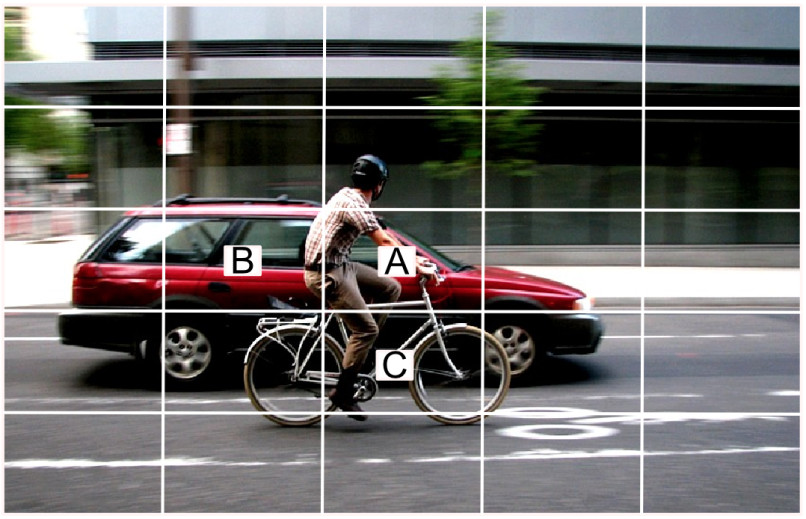Image B

We can notice that the cell C will be the one that detects the bicycle, the cell A will be the one that detects the person and the cell B will be the one that detects the car.

then the arrays for these cells could be like:

``````
cell_a = [0.8, 0.14, 0.15, 0.54, 0.97, 0.734, 0.24, 0.026]

cell_b = [0.7, 0.04, 0.05, 0.94, 0.97, 0.24, 0.734, 0.026]

cell_c = [0.95, 0.14, 0.15, 0.84, 0.87, 0.034, 0.032, 0.934]

``````

In a next post we will use a one-shot detector to detect pneumonia in lungs images, in that project we will use an array of 5 values for each cell where: [cs, x_min, y_min, x_max, y_max], since we will only have two classes we can use the confidence score instead of class values, if the confidence score is greater than 0.5 then the predicted class would be the class 1, otherwise the predicted class would be the class 0.

Before moving on we have to know two things, the first one is that we can have multiple detectors in each cell, for example we could have 75 detectors instead of 25, to obtain 75 detectors we will need 3 detectors in each cell, consequently each array will have a size of 24:

[cs, x_min, y_min, x_max, y_max, class1, class2, class3, cs, x_min, y_min, x_max, y_max, class1, class2, class3, cs, x_min, y_min, x_max, y_max, class1, class2, class3]

5x5x3 = 75

and the feature map size would be of 5x5x24, in fact the idea behind multiple detectors for each cell is that each detector has a different shape and size: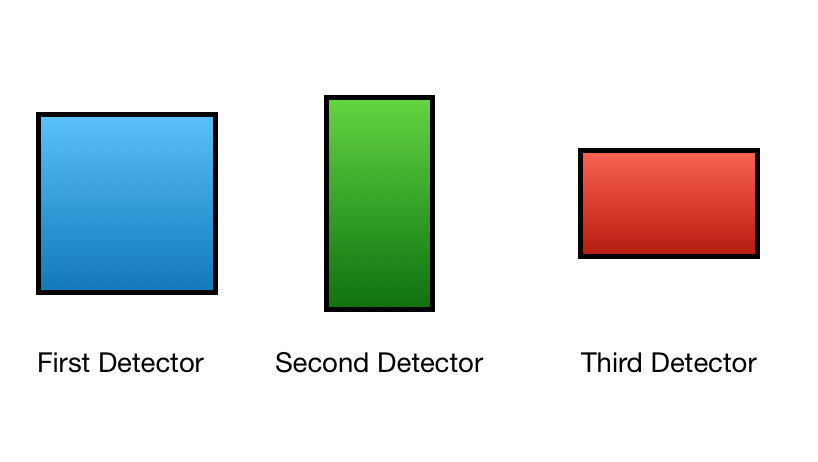Image C

For example if one cell detects a car the third detector would fit better the car object, if the cell detects a person the second detector would fit better the person object, another advantage is that now each cell can detect multiple objects and not only one. We adjust the size of each detector when we are building the y_train and y_val variables.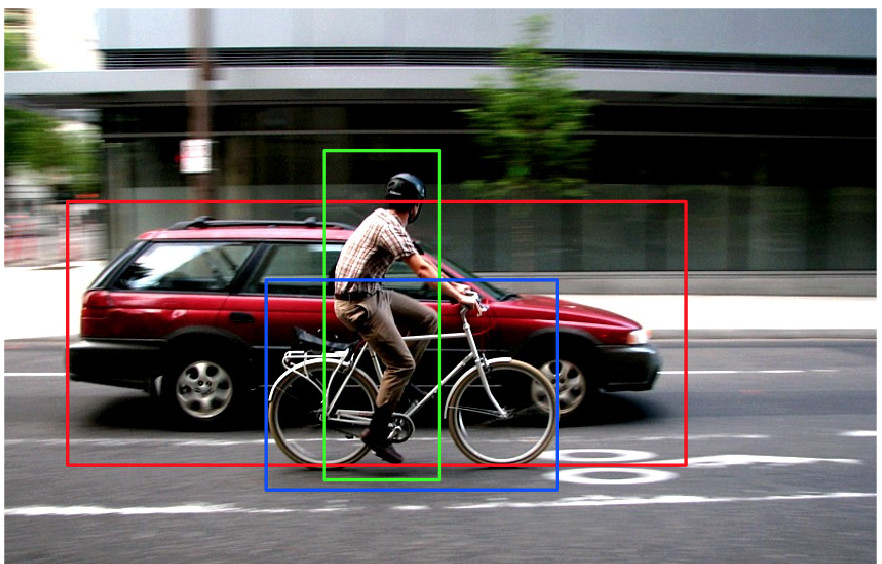Image D

The second thing we should know is that we can change the number of cells of the feature map, actually is pretty common have multiple feature maps with different number of cells, for example in the image B we can notice that the objects are quite big and do not fit well in the cells, we can solve this using less cells:Image E

Now we only have 9 cells, we can notice that the cell C can detect the bycicle and the cell B|A as we learned in the image D can detect the two remaining objects. For instane if we have big objects in the images we should add feature maps with less cells and if we have small objects we should add feature maps with more cells.

In TensorFlow we can code this like:

``````
first_feature_map = Conv2D(5, 3, padding='valid', activation='sigmoid')(mobile_model_output)

second_feature_map = Conv2D(10, 4, padding='valid', activation='sigmoid')(mobile_model_output)

third_feature_map = Conv2D(20, 5, padding='valid', activation='sigmoid')(mobile_model_output)

``````

We have to change the number of kernels to add more detectors and change the size of these kernels to obtain more or fewer cells.

As I mentioned before In the next tutorial we will only use one feature map of size 5x5x5 with one detector for each cell.

## The Volume Tensor

Now that we understand how the cells and the detectors work we can build our label variables, to achieve this we need to build a tensor like the output feature map and localize the coordinates of each object.

If we want to build an object detection network our dataset must have the coordinates of the objects that appear in the images.

We have to create a tensor of size 5x5x5 for each image, therefore we will obtain a tensor of size mx5x5x5 where m is the number of images in the dataset, we call this tensor the volume tensor.

We need a for loop to go through all the images:

``````
tensor_volume = []

for image in images:

boxes = image.coords

volume = create_volume(boxes)

tensor_volume.append(volume)

``````

In the code above we obtain the coordinates of each object in each image, the image can contain none or multiple objects, the volume variable is a tensor of size 5x5x5 which we will use to save the locations of the objects in one image.

``````
def create_volume(boxes):

grid_volume = np.zeros((5, 5, 5))

for box in boxes:

if max(box) == 0:

continue

_, (column, row) = get_anchor(box)

grid_volume[column, row, :] = [1, *box]

return grid_volume

``````

The create_volume function receives the boxes (coordinates) of the objects in the image, grid_volume is the tensor of size 5x5x5 but only filled of zeros, if we print this variable we will see the following tensor:

``````
[[[0., 0., 0., 0., 0.],

[0., 0., 0., 0., 0.],

[0., 0., 0., 0., 0.],

[0., 0., 0., 0., 0.],

[0., 0., 0., 0., 0.]],

[[0., 0., 0., 0., 0.],

[0., 0., 0., 0., 0.],

[0., 0., 0., 0., 0.],

[0., 0., 0., 0., 0.],

[0., 0., 0., 0., 0.]],

[[0., 0., 0., 0., 0.],

[0., 0., 0., 0., 0.],

[0., 0., 0., 0., 0.],

[0., 0., 0., 0., 0.],

[0., 0., 0., 0., 0.]],

[[0., 0., 0., 0., 0.],

[0., 0., 0., 0., 0.],

[0., 0., 0., 0., 0.],

[0., 0., 0., 0., 0.],

[0., 0., 0., 0., 0.]],

[[0., 0., 0., 0., 0.],

[0., 0., 0., 0., 0.],

[0., 0., 0., 0., 0.],

[0., 0., 0., 0., 0.],

[0., 0., 0., 0., 0.]]]

``````

If we would print the output feature map as well, we would obtain a similar tensor but with the predictions for each cell, for example if our network found out an object in the cell [3, 2] row 2 and column 1, we could print its coordinates:

``````
grid_volume

``````

we would obtain an array like:

``````
[0.8, 0.14, 0.15, 0.54, 0.97]

``````

In the for loop firstly we check if the boxes have real coordinates, as I mentioned before the image can contain none or multiple objects, if the image has no objects then the boxes variable is an array full of zeros:

``````
[[0, 0, 0, 0, 0]]

``````

If this is the case then we will return the grid_volume tensor only filled of zeros to indicate that the image does not contains objects, if the image contains one object the boxes variable would be:

``````
[[0.8, 0.14, 0.15, 0.54, 0.97]]

``````

whereas if the image contains multiple objects then the variable would be:

``````
[[0.8, 0.14, 0.15, 0.54, 0.97], [0.8, 0.14, 0.15, 0.54, 0.97], [0.8, 0.14, 0.15, 0.54, 0.97]]

``````

The for loop after checking that the box contains real coordinates will execute the function get_anchor, this function returns the number of column and row where the object is located, once we have the location we can fill the array with the object's coords:

``````
grid_volume[column, row, :] = [1, *box]

``````

Where row is 1 and column is 2

and now our grid_volume variable has these coords saved:

``````
[[[0.  , 0.  , 0.  , 0.  , 0.  ],

[0.  , 0.  , 0.  , 0.  , 0.  ],

[0.  , 0.  , 0.  , 0.  , 0.  ],

[0.  , 0.  , 0.  , 0.  , 0.  ],

[0.  , 0.  , 0.  , 0.  , 0.  ]],

[[0.  , 0.  , 0.  , 0.  , 0.  ],

[0.  , 0.  , 0.  , 0.  , 0.  ],

[1 , 0.14, 0.15, 0.54, 0.97], # righ here

[0.  , 0.  , 0.  , 0.  , 0.  ],

[0.  , 0.  , 0.  , 0.  , 0.  ]],

[[0.  , 0.  , 0.  , 0.  , 0.  ],

[0.  , 0.  , 0.  , 0.  , 0.  ],

[0.  , 0.  , 0.  , 0.  , 0.  ],

[0.  , 0.  , 0.  , 0.  , 0.  ],

[0.  , 0.  , 0.  , 0.  , 0.  ]],

[[0.  , 0.  , 0.  , 0.  , 0.  ],

[0.  , 0.  , 0.  , 0.  , 0.  ],

[0.  , 0.  , 0.  , 0.  , 0.  ],

[0.  , 0.  , 0.  , 0.  , 0.  ],

[0.  , 0.  , 0.  , 0.  , 0.  ]],

[[0.  , 0.  , 0.  , 0.  , 0.  ],

[0.  , 0.  , 0.  , 0.  , 0.  ],

[0.  , 0.  , 0.  , 0.  , 0.  ],

[0.  , 0.  , 0.  , 0.  , 0.  ],

[0.  , 0.  , 0.  , 0.  , 0.  ]]]

``````

We must notice that we use the number 1 at the beginning of the array to indicate that the cell contains an object.

Now we will see the get_anchor function which does the hard work:

``````
grid_size = 5

def get_anchor(box):

max_iou = 0.0

best_anchor = [0, 0, 0, 0]

best_anchor_index = (0, 0)

column = 0

row = 0

cell_width, cell_height = (1 / grid_size, 1 / grid_size)

for cell_x_position in np.linspace(0, 1, grid_size + 1)[:-1]:

row = 0

for cell_y_position in np.linspace(0, 1, grid_size + 1)[:-1]:

x_min = cell_x_position

y_min = cell_y_position

x_max = (cell_x_position + cell_width)

y_max = (cell_y_position + cell_height)

anchor_box = [x_min, y_min, x_max, y_max]

current_iou = iou(box, anchor_box)

if current_iou > max_iou:

best_anchor = anchor_box

max_iou = current_iou

best_anchor_index = (column, row)

row += 1

column += 1

return best_anchor, best_anchor_index

``````

This function has several important parts:

Since our grid_volume has a min value of 0 and a max value of 1 all our objects will have coordinates between 0 and 1, in order to get the coordinates of each object in this grid we have to obtain the coordinates of each cell, we can achieve this with the variables cell_x_position, cell_y_position and cell_width, cell_height, we know that our grid_volume has 5x5 cells, then cell_x_position and cell_y_position will take the values [0, 0.2, 0.4, 0.6, 0.8] and each cell has a size of 0.2 (1/5 = 0.2) for instance if we are in the cell [2, 3] row 1 and column 2:

• cell_x_position = 0.4

• cell_y_position = 0.2

Consequently:

``````
x_min = 0.4

y_min = 0.2

x_max = (0.4 + 0.2) #0.6

y_max = (0.2 + 0.2) #0.4

``````

Then we build a variable with these coordinates:

``````
anchor_box = [x_min, y_min, x_max, y_max]

``````

Finally we need to know if our current cell is good enough to fit the object:

``````
current_iou = iou(box, anchor_box)

``````

## Intersection Over Union

The iou function uses a method called intersection over union to check the match between two boxes:

``````
def iou(box, anchor_box):

x_min = np.maximum(box, anchor_box)

y_min = np.maximum(box, anchor_box)

x_max = np.maximum(box, anchor_box)

y_max = np.maximum(box, anchor_box)

overlap_area = np.maximum(0.0, x_max - x_min + 1) * np.maximum(0.0, y_max - y_min + 1)

true_boxes_area = (box - box + 1) * (box - box + 1)

anchor_boxes_area = (anchor_box - anchor_box + 1) * (anchor_box - anchor_box + 1)

union_area = (true_boxes_area + anchor_boxes_area - overlap_area)

return overlap_area / union_area

``````

In this function we calculate the area of overlap: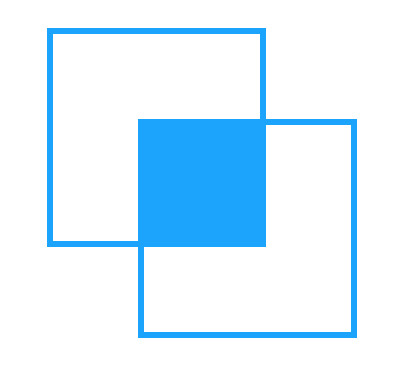Which is the area where both boxes match

And we calculate the area of union:Which is the total area of both boxes.

We divide the area of union by the area of overlap to obtain the intersection over union value, If both boxes have the same coordinates then this value is 1, therefore we will look for the cell which gives us the maximum intersection over union value.

If we would be using more detectors we compute each detector's coordinates with respect to its cell, for example if we have a detector which is wider than tall and the cell coordinates are:

``````
x_min = 0.4

y_min = 0.2

x_max = 0.6

y_max = 0.4

``````

the detector could have the following coordinates:

``````
x_min = 0.4

y_min = 0.25

x_max = 0.6

y_max = 0.35

``````

then we would use the detectors in the iou function to see which detector fits better the object.

To sum up we will move through each cell, obtain its coordinates and compare this coordinates with the object's coordinates, once we find out the cell with the maximum intersection over union value we will use this cell as the object location.

## Loss function

We need a custom loss function in order to compute the object detection loss and the class prediction loss, this loss function will receive the tensor volume than we created in the previous section (y_true) and the output feature map from the model (y_pred):

``````
def custom_loss(y_true, y_pred):

mask = y_true[..., 0]

detection_loss = tf.losses.absolute_difference(true_boxes[..., 1:], predicted_boxes[..., 1:])

prediction_loss = tf.keras.losses.binary_crossentropy(y_true[..., 0], y_pred[..., 0])

return tf.reduce_mean(prediction_loss) + 10 * detection_loss

``````

We want to know how good the model is predicting the coordinates of each object, therefore we only take into account the cells which contain objects.

Firstly we will obtain these cells:

``````
mask = y_true[..., 0]

``````

With the tf.boolean_mask function we can find out the cells that contains the number 1 in the first position of the array, as we know this number 1 indicates that the cell contains an object.

Now to compute the detection loss we need the coordinates:

``````
detection_loss = tf.losses.absolute_difference(true_boxes[..., 1:], predicted_boxes[..., 1:])

``````

Finally in order to check the prediction loss we need the class values and we know that these are in the first position of each cell:

``````
prediction_error = tf.keras.losses.binary_crossentropy(y_true[..., 0], y_pred[..., 0])

``````

As you can notice to compute the prediction loss we use all the cells even if they do not have objects.

The final step is train the model, first we need to compile the model and indicate our loss function:

``````
model.compile(loss=custom_loss, metrics=[custom_accuracy, mean_iou], optimizer=optimizer)

``````

Using a custom generator we can train the model:

``````
trained_model = model.fit_generator(train_generator,

epochs=epochs,

steps_per_epoch=train_steps,

validation_data=val_generator,

validation_steps=val_steps,

verbose=1)

``````

You can see the complete code in this notebook, in the notebook you can find out how to create a custom generator, how to plot the true boxes and the predicted boxes along with the images, how to use a custom accuracy and use the intersection over union function as a metric.

The code of the notebook is based on the code of this notebook, actually the author of this last notebook has a lot of excellent notebooks, I reuse a lot of the functions but I changed the name of some variables and functions to make the code easier to read.

In fact I made this post since I wanted to explain how One-shot detectors work, as you can notice in the notebook I used the one-shot detector to detect lung opacities in some areas of the lungs, therefore I needed a neural network capable of predict coordinates. In my next post I will talk about this project.